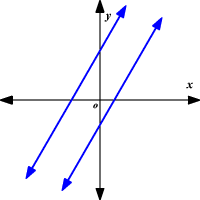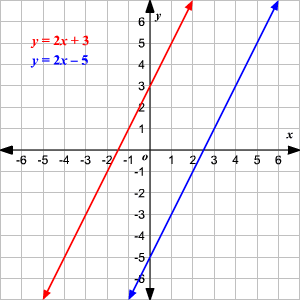# Parallel Lines and Slopes

Parallel lines are coplanar lines that do not intersect. In two dimensions, parallel lines have the same slope .We can write the equation of a line parallel to a given line if we know a point on the line and an equation of the given line.

Example:

Write the equation of a line that passes through the point $\left(3,1\right)$ and is parallel to the line

$y=2x+3$ .

Parallel lines have the same slope.

The slope of the line with equation $y=2x+3$ is $2$ . So, any line parallel to $y=2x+3$ has the same slope $2$ .

Now use the point-slope form to find the equation.

$y-{y}_{1}=m\left(x-{x}_{1}\right)$

We have to find the equation of the line which has slope $2$ and passes through the point $\left(3,1\right)$ . So, replace $m$ with $2$ , ${x}_{1}$ with $3$ , and ${y}_{1}$ with $1$ .

$y-1=2\left(x-3\right)$

Use the distributive property .

$y-1=2x-6$

Add $1$ to each side.

$\begin{array}{l}y-1+1=2x-6+1\\ \text{\hspace{0.17em}}\text{\hspace{0.17em}}\text{\hspace{0.17em}}\text{\hspace{0.17em}}\text{\hspace{0.17em}}\text{\hspace{0.17em}}\text{\hspace{0.17em}}\text{\hspace{0.17em}}\text{\hspace{0.17em}}\text{\hspace{0.17em}}\text{\hspace{0.17em}}\text{\hspace{0.17em}}\text{\hspace{0.17em}}y=2x-5\end{array}$

Therefore, the line $y=2x-5$ is parallel to the line $y=2x+3$ and passes through the point $\left(3,1\right)$ .